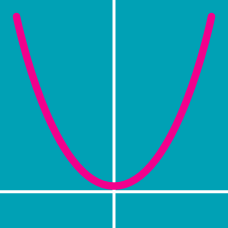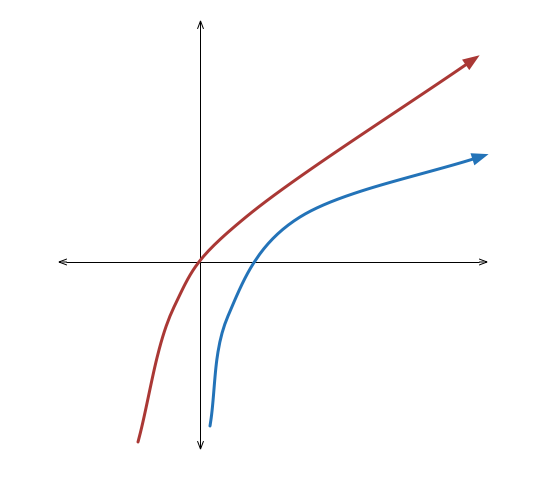Algebra

# Function Graphs: Level 2 Challenges

$\large (X-1)(X-3)(X-5)(X-7)\ldots (X-97) < 0$

How many positive integers $X$ satisfy the inequality above?

Determine whether the function $f(x) = \ln(x+ \sqrt{1+x^2} )$ is an odd function or an even function.

$\left| 1-\left| 1-\left| x-1 \right| \right| \right| =\alpha$

Find the value of $\alpha$ for which this equation has exactly 4 distinct solutions. If the value of $\alpha$ is $A$, then find $2A$.

Find the area of the region bound by the equation $|x+2y|+|2x-y|=10$Given the graph $y = \ln x$, which of these statements describes the transformations to get the graph of $y = \ln (4x^2 + 4x + 1)$ for $x > - \frac{1}{2}$?

$\quad \text{(1)}$ Translate to the left by 1 and up by $\ln 4$, then scale vertically by 2.

$\quad \text{(2)}$ Translate to the left by $\frac{1}{2}$ and up by $\ln 2$, then scale vertically by 2.

$\quad \text{(3)}$ Translate to the left by 1 and up by $\ln 2$, then scale vertically by 2.

$\quad \text{(4)}$ Translate to the left by $\frac{1}{2}$ and up by $\ln 4$, then scale vertically by 2.

×